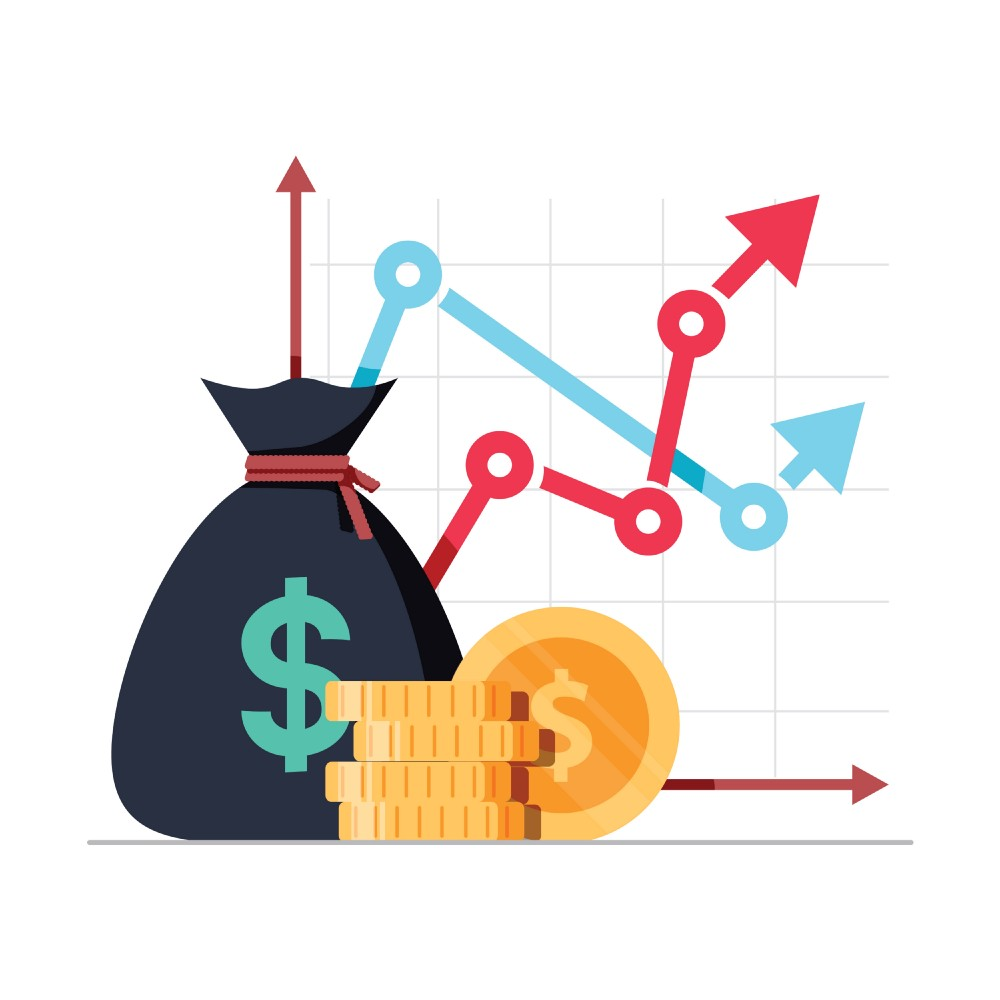# The Loan ConstantChris PetersMost real estate investors utilize leverage to increase returns and as a financing platform, our job is to source, structure and negotiate that leverage. When it comes to leverage, investors should be aware of how the cost and amount of leverage can affect an investment’s performance. One way to do that is to compare the cap rate to the loan constant.

As we know, the cap rate can be thought of as the initial yield on an investment (note: this only applies to stabilized investment deals, because a cap rate is only a spot measure). A property with a 7% cap rate is essentially yielding 7% (unlevered) if stabilized.

The loan constant, also referred to as the mortgage constant, is a metric used to determine the total amount of debt service paid on a loan balance. It goes one step beyond the interest rate by incorporating the repayment of principal on an amortizing loan and is calculated as the total annual debt service (principal and interest) divided by the loan amount. See an example below:

If we have a \$1,000,000 loan, a 5% interest rate and a 25 year amortization schedule, the annual debt service is \$70,151 and the loan constant is calculated as \$70,151 / \$1,000,000 = 7.02%. If we increase the amortization schedule to 30 years (pay back the principal over a longer period of time), the annual debt service is \$64,419 and the loan constant drops to 6.44%.

This number, expressed as a percentage, takes into account the repayment of principal and interest whereas the interest rate simply takes into account interest payments.

So, how do we analyze the relationship between the cap rate and loan constant? When comparing the two, investors should be aware of which number is greater.

For example, with a cap rate of 7% and a loan constant of 6%, there is more income than debt service which causes positive leverage. If an investor were to increase leverage from 50% to 80%, they should see their return on equity (cash on cash) increase.

Conversely, with a loan constant of 7% and a cap rate of 6%, there is more debt service than income which causes negative leverage. If an investor were also to increase leverage from 50% to 80%, they would see their return on equity decrease.

Investors need to track this relationship and how it affects their investment’s return profile. Increasing leverage when the cap rate is greater than the loan constant will further increase returns, however increasing leverage when the cap rate is less than the loan constant will decrease returns.

So, for stabilized investment deals, it’s good practice for investors to first determine the loan constant, and then compare it to the cap rate, and increase leverage when there is positive leverage and decrease leverage when there is negative leverage.StackSource is a tech-enabled commercial real estate loan platform. We connect investors who are developing or acquiring commercial properties with financing options like banks, insurance companies, and debt funds through a transparent online process. We’re taking the best of commercial mortgage brokerage and updating it for the 21st century. Learn more at StackSource.com.